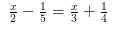# Solve the following linear equations: x/2 - 1/5 = x/3 + 1/4

Solve the following linear equations: